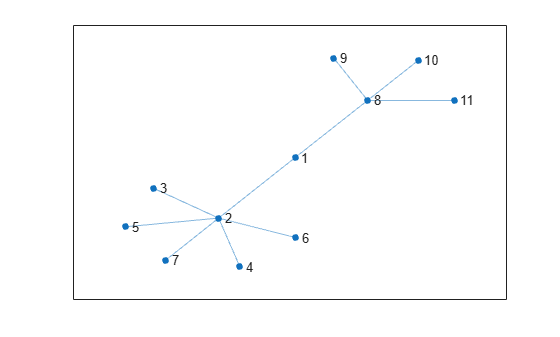subgraph

Extract subgraph

Description

example

H = subgraph(G,nodeIDs) returns a subgraph of G that contains only the nodes specified by nodeIDs.

H = subgraph(G,idx) specifies the subgraph nodes using a logical vector.

Examples

collapse all

Create and plot a graph.

s = [1 1 1 1 2 2 2 2 2 2 2 2 2 2 15 15 15 15 15];
t = [3 5 4 2 14 6 11 12 13 10 7 9 8 15 16 17 19 18 20];
G = graph(s,t);
plot(G,'Layout','force')Extract a subgraph from G by specifying which nodes to include. The node numbering in the subgraph is reset.

idx = [2 15 16 17 18 19 20 1 3 4 5];
H = subgraph(G,idx);
plot(H,'Layout','force')Create and plot a weighted graph with named nodes.

s = [1 1 1 2 2 2 8 8 8 8];
t = [2 3 4 5 6 7 9 10 11 12];
weights = [10 30 40 80 60 60 20 30 90 80];
names = {'A' 'B' 'C' 'D' 'E' 'F' 'G' 'H' 'I' 'J' 'K' 'L'};
G = graph(s,t,weights,names);
plot(G,'EdgeLabel',G.Edges.Weight)Extract a subgraph that contains node 'B' and all of its neighbors. subgraph preserves the node names and edge weights. However, the numeric node IDs in H are renumbered compared to G.

N = neighbors(G,'B');
H = subgraph(G, ['B'; N]);
plot(H,'EdgeLabel',H.Edges.Weight)Input Arguments

collapse all

Input graph, specified as either a graph or digraph object. Use graph to create an undirected graph or digraph to create a directed graph.

Example: G = graph(1,2)

Example: G = digraph([1 2],[2 3])

Node identifiers, specified as one or more node indices or node names. nodeIDs selects a subset of the nodes in G to generate the subgraph, H.

This table shows the different ways to refer to one or more nodes either by their numeric node indices or by their node names.

FormSingle NodeMultiple Nodes
Node index

Scalar

Example: 1

Vector

Example: [1 2 3]

Node name

Character vector

Example: 'A'

Cell array of character vectors

Example: {'A' 'B' 'C'}

String scalar

Example: "A"

String array

Example: ["A" "B" "C"]

Example: H = subgraph(G,[1 2 5])

Example: H = subgraph(G,{'A' 'B' 'E'})

Node selection vector, specified as a logical vector. The subgraph contains only the nodes J for which idx(J) is logical 1 (true). The index of node J in H is I(J), where I = find(idx).

Example: subgraph(G,degree(G)>2)

Data Types: logical

Output Arguments

collapse all

Subgraph, returned as a graph or digraph object. H contains only the nodes that were selected with nodeIDs or idx. Other nodes in G (and the edges connecting to those nodes) are discarded. The node properties and edge properties of the selected nodes and edges are carried over from G into H.